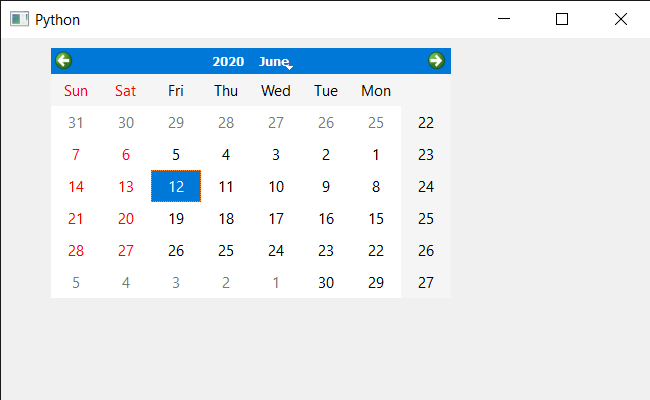Open in App
Not now

# PyQt5 QCalendarWidget – Setting Change Event

• Last Updated : 27 Jan, 2022

In this article we will see how we can implement the change event for the QCalendarWidget. This event handler can be reimplemented to handle state changes. The state being changed in this event can be retrieved through the event supplied. State change can be the layout direction, language change etc.

Below is the Calendar class code

```# QCalendarWidget Class
class Calendar(QCalendarWidget):

# constructor
def __init__(self, parent=None):
super(Calendar, self).__init__(parent)

# overriding the changeEvent method
def changeEvent(self, event):

# setting text to the label
print("Change Event Called")

```

Implementation steps:
1. Create a Calendar class that inherits the QCalendarWidget
2. Inside the Calendar class override the changeEvent and inside the event print the text
3. Create a main window class
4. Create a Calendar object inside the main window
5. Set various properties to the calendar
6. Change the direction of the calendar

Below is the implementation

## Python3

 `# importing libraries``from` `PyQt5.QtWidgets ``import` `*` `from` `PyQt5 ``import` `QtCore, QtGui``from` `PyQt5.QtGui ``import` `*` `from` `PyQt5.QtCore ``import` `*` `import` `sys`` ` `# QCalendarWidget Class``class` `Calendar(QCalendarWidget):`` ` `    ``# constructor``    ``def` `__init__(``self``, parent ``=` `None``):``        ``super``(Calendar, ``self``).__init__(parent)`` ` `    ``# overriding the changeEvent method``    ``def` `changeEvent(``self``, event):`` ` `        ``# setting text to the label``        ``print``(``"Change Event Called"``)`` ` ` ` `class` `Window(QMainWindow):`` ` `    ``def` `__init__(``self``):``        ``super``().__init__()`` ` `        ``# setting title``        ``self``.setWindowTitle(``"Python "``)`` ` `        ``# setting geometry``        ``self``.setGeometry(``100``, ``100``, ``650``, ``400``)`` ` `        ``# calling method``        ``self``.UiComponents()`` ` `        ``# showing all the widgets``        ``self``.show()`` ` `    ``# method for components``    ``def` `UiComponents(``self``):`` ` `        ``# creating a QCalendarWidget object``        ``# as Calendar class inherits QCalendarWidget``        ``self``.calendar ``=` `Calendar(``self``)`` ` `        ``# setting geometry to the calendar``        ``self``.calendar.setGeometry(``50``, ``10``, ``400``, ``250``)`` ` `        ``# setting cursor``        ``self``.calendar.setCursor(Qt.PointingHandCursor)`` ` `        ``# setting direction right to left``        ``self``.calendar.setLayoutDirection(``1``)`` ` ` ` ` ` ` ` `# create pyqt5 app``App ``=` `QApplication(sys.argv)`` ` `# create the instance of our Window``window ``=` `Window()`` ` `# start the app``sys.exit(App.``exec``())`

Output :

`Change Event Called`My Personal Notes arrow_drop_up